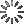• Share
• Login

# Javascript String ComparisonRishabh

String Comparison is an important thing to understand in Javascript. We need to make sure that we comprehend how string comparison works exactly with different operators like `<` `<=` `>` `>=` `===` `==`.

• `<` stands for less than. So `a < b` checks whether `a` is less than `b` or not, and if that's true, then it evaluates to true, else it evaluates to false.
• `<=` means less than OR equal to. So `a <= b` checkes whether `a` is less than OR equal to `b`. If any of those are true, then it evaluates to true. Else it evaluates to false.
• `>` and `>=` are similar to the above examples, but in the reverse order.
• `===` checks whether 2 operands are strictly equal or not. So, `a === b` checks whether `a` and `b` are strictly equal or not. By strictly, I mean, their types (strings, numbers, etc.) should be same and the values should also be same. It is recommended to use `===` instead of `==` because `==` does some conversion (type coercion) when the 2 operands are of different types, causing weird bugs. So `1234 == '1234'` is true while `1234 === '1234'` evaluates to false.

This understanding will help us write our programs better, and make sure that we don't produce bugs by using the incorrect operator.

Note: String comparison is based on ASCII. Here's the ASCII Table: http://www.asciitable.com/

#### Related:

Warning: Invalid argument supplied for foreach() in /var/www/cssdeck/app/modules/labs/views/_details.php on line 68

### Validations

(Errors from your JS or Pre-Processors code)

HTML
CSS
JavaScript

CSSDeck G+